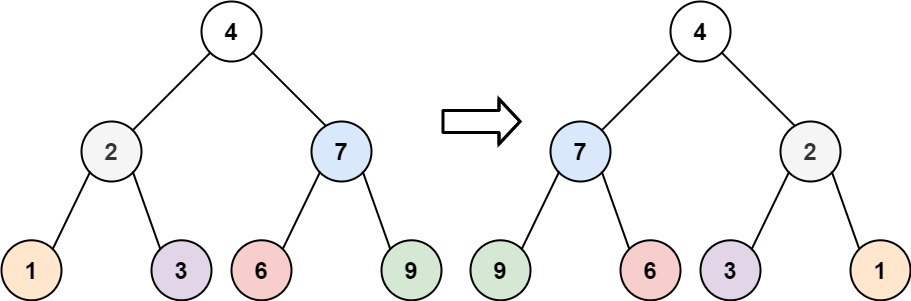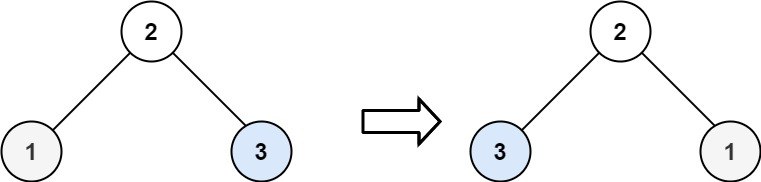304 North Cardinal St.
Dorchester Center, MA 02124

# Invert Binary Tree LeetCode Solution

## Problem – Invert Binary Tree LeetCode Solution

Given the `root` of a binary tree, invert the tree, and return its root.

Example 1:``````Input: root = [4,2,7,1,3,6,9]
Output: [4,7,2,9,6,3,1]
``````

Example 2:``````Input: root = [2,1,3]
Output: [2,3,1]
``````

Example 3:

``````Input: root = []
Output: []
``````

Constraints:

• The number of nodes in the tree is in the range `[0, 100]`.
• `-100 <= Node.val <= 100`

## Invert Binary Tree LeetCode Solution in Python

``````def invertTree(self, root):
if root:
root.left, root.right = self.invertTree(root.right), self.invertTree(root.left)
return root
``````

## Invert Binary Tree LeetCode Solution in C++

``````TreeNode* invertTree(TreeNode* root) {
if (root) {
invertTree(root->left);
invertTree(root->right);
std::swap(root->left, root->right);
}
return root;
}
``````

## Invert Binary Tree LeetCode Solution in Java

``````public class Solution {
public TreeNode invertTree(TreeNode root) {
if(root == null) return null;
TreeNode tmp = root.left;
root.left = invertTree(root.right);
root.right = invertTree(tmp);
return root;
}
}
``````
##### Invert Binary Tree LeetCode Solution Review:

In our experience, we suggest you solve this Invert Binary Tree LeetCode Solution and gain some new skills from Professionals completely free and we assure you will be worth it.

If you are stuck anywhere between any coding problem, just visit Queslers to get the Invert Binary Tree LeetCode Solution

Find on Leetcode

##### Conclusion:

I hope this Invert Binary Tree LeetCode Solution would be useful for you to learn something new from this problem. If it helped you then don’t forget to bookmark our site for more Coding Solutions.

This Problem is intended for audiences of all experiences who are interested in learning about Data Science in a business context; there are no prerequisites.

Keep Learning!

More Coding Solutions >>

LeetCode Solutions

Hacker Rank Solutions

CodeChef Solutions